Fractions - examples - page 12

1. DoublingThe message is spreading that each day has doubled the number of people who know about it. All know message for 20 days. How long known it eighth people?
2. Oceans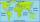The Earth's surface is approximately 510,000,000 km2 and is 7/10 covered by oceans. Of which 1/2 covers the Pacific Ocean, the Atlantic Ocean 1/4, the Indian Ocean 1/5 and the Arctic Ocean 1/20. What parts of the Earth's surface cover each ocean?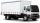How many products weighing 12.5 kg can be loaded on a cargo car with a load of 1.5 t to load two-thirds?
4. Package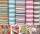The package was 23 meters of textile. The first day sold 12.3 meters. How many meters of textile remained in the package?
5. Two sisters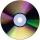Two sisters together have 54 CDs. 6/8 CDs has younger sister and is equal to 3/5 of CDs older sisters. How many CDs has each of the sisters?
6. ClassroomOne-eighth of 9th class was interested in studying at a grammar school, at a business academy one sixth, at secondary vocational schools quarter, to SOU one third and the remaining three students were interested in the school of art direction. How many st
7. Ten fractionsWrite ten fractions between 1/3 and 2/3
8. DividendsThe three friends divided the win by the invested money. Karlos got three eighths John 320 permille and the rest got Martin. Who got the most and which the least?
9. ChocolateChildren break chocolate first to third and then every part of another half. What kind got each child? Draw a picture. What part would have received if each piece have halved?
10. Unknown number 6Determine x if 1/6 of x is equal to 2/5 of the number 24.
11. Rolls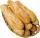Mom bought 13 rolls. Dad ate 3.5 rolls. How many rolls left when Peter yet put two at dinner?
12. CandiesThere are red, blue and green candies in bad. Red to green is in 6:11 ratio and blue to red in a 7: 5 ratio. In what proportion are blue to green candies?
13. The bunsKate, Zofia and Peter Liked buns. Even today, their grandmother prepare their favorite meal. Katka eats 4 bunches, Žofia 3 and Petra eats 5 buns. Their grandmother said to them, "My inmate will you know how many buns I have been make today, if those you ea
14. Guess a fractionTom was asked to guess a fraction. The sum of 1/2 the numerator and 1/3 of its denominator is 30. If Tom subtracts 36 from its denominator, the fraction becomes 1/3. What is the fraction that Tom was asked to guess? (Leave your answer in simplest form)
15. Ratio - proportion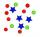Reduce the number 16 in proportion 3:2 5:4 11:8
16. LCD 2The least common denominator of 2/5, 1/2, and 3/4
17. Unknown number 23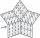Find 2/3 of unknown number, which is two thirds of the 99.
18. Bar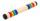Half of the bar is green, the third is yellow and the remaining 40cm is red. How long is a bar?
19. Cleaning windowsCleaning company has to wash all the windows of the school. The first day washes one-sixth of the windows of the school, the next day three more windows than the first day and the remaining 18 windows washes on the third day. Calculate how many windows ha
20. Red ballsIn the bag there are 3 red, 12 blue and 8 green balls. How many red balls we must be attached to the bag if we want the probability of pulling out the red balls was 20%?

Do you have an interesting mathematical example that you can't solve it? Enter it, and we can try to solve it.

To this e-mail address, we will reply solution; solved examples are also published here. Please enter e-mail correctly and check whether you don't have a full mailbox.

Need help calculate sum, simplify or multiply fractions? Try our fraction calculator.